# Count of roots

How many solutions has equation x. y = 7757 with two unknowns on the set of natural numbers?

Result

n =  2

#### Solution:Leave us a comment of example and its solution (i.e. if it is still somewhat unclear...):

Showing 0 comments:Be the first to comment!## Next similar examples:

1. Divisibility 2How many divisors has integer number 13?
2. Digit sumDetermine for how many integers greater than 900 and less than 1,001 has digit sum digit of the digit sum number 1.
3. Tailor master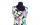There are less than 50m of textile in the tailoring workshop. When cutting on a blouse (consumption 1.5m), no textile is left. When using a cloth (consumption of 3.2m), no textile is left. How many meters of textile are in a tailor's workshop?
4. PlumsIn the bowl are plums. How many would be there if we can divide it equally among 8, 10 and 11 children?
5. Trams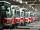Tram no. 3,7,10,11 rode together from the depot at 5am. Tram No. 3 returns after 2 hours, tram No. 7 an hour and half, no. 10 in 45 minutes and no. 11 in 30 minutes. For how many minutes and when these trams meet again?
6. DisjointHow many elements have union and intersection of two disjoint sets when the first have 1 and secodn 8 elements.
7. Troops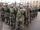If the general sorts troops into the crowd by nine left 6. How many soldiers has regiment if we know that they are less than 300?
8. Lines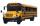Five bus lines runs at intervals 3, 6, 9, 12, 15 minutes. In the morning, suddenly start at 4:00. After how many minutes the bus lines meet again?
9. Game roomWinner can took win in three types of jettons with value 3, 30 and 100 dollars. What is minimal value of win payable in this values of jettons?
10. Street numbersLada came to aunt. On the way he noticed that the houses on the left side of the street have odd numbers on the right side and even numbers. The street where he lives aunt, there are 5 houses with an even number, which contains at least one digit number 6.
11. Two-digit numberI am a two-digit number less than 20. When I divided by three, then you get the rest 1 and when you divide me by four you get also rest 1. What number am I?
12. Prime factorsWrite 98 as product of prime factors
13. DivisibilityDetermine all divisors of number 84.
14. Write decimalsWrite in the decimal system the short and advanced form of these numbers: a) four thousand seventy-nine b) five hundred and one thousand six hundred and ten c) nine million twenty-six
15. Lcm 2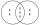Create the smallest possible number that is divisible by numbers 5,8,9,4,3
16. LCMWhat is the least common multiple of 36, 44, 21?
17. Decomposition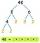Make decomposition using prime numbers of number 155. Result write as prime factors (all, even multiple)